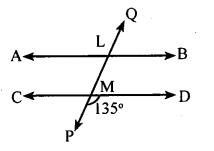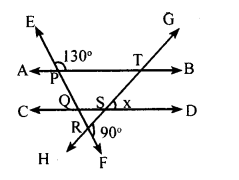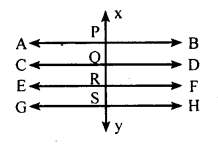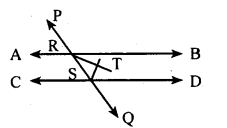# KSEEB Solutions for Class 8 Maths Chapter 3 Axioms, Postulates and Theorems Ex 3.3

Students can Download Maths Chapter 3 Axioms, Postulates and Theorems Ex 3.3 Questions and Answers, Notes Pdf, KSEEB Solutions for Class 8 Maths helps you to revise the complete Karnataka State Board Syllabus and score more marks in your examinations.

## Karnataka Board Class 8 Maths Chapter 3 Axioms, Postulates and Theorems Ex 3.3

Question 1.
Find all the angles in the following∠CMP + ∠PMD = 180° [Linear pair]
∠CMP+ 135° = 180°
∠CMP = 180° – 135
∠CMP = 45°
∠LMD = ∠CMP = 45° [Vertical opposite angles]
∠LMC =∠PMD = 135° [Vertical opposite angles]
∠ALM = ∠ LMC = 135° [Alternate angles]
∠BLM = ∠ LMC = 135° [Alternate angle]
∠QLB = ∠LMD = 45° [Corresponding angles]
∠QLA = ∠ LMC = 135° [Corresponding angles

Question 2.
Find the value of x in the diagram below.∠PQS = ∠EPT = 130° [Corresponding angle]
∠PQS + ∠SQR = 180° [Linear pair]
SQR = 180 – 130
∠SQR = 50°
∠QRS + ∠FRS = 180° [Linear pair]
∠QRS + 90° =180°
∠QRS = 180 – 90
∠QRS = 90°
∠SQR+ ∠QRS +∠QSR = 180°
[Sum of the angles of triangle is 180° ]
50 + 90 + ∠QSR = 180°
140 + ∠QSR = 180°
∠QSR = 180 – 140
∠QSR =40°
∠TSD = ∠QSR [Vertically opposite angles]
x = 40°.

Question 3.
Show that if a straight line is perpendicular to one of the two or more parallel lines, then it is also perpendicular to the remaining lines.$$\overrightarrow{\mathrm{AB}}\|\overrightarrow{\mathrm{CD}}\| \overrightarrow{\mathrm{EF}} \| \overrightarrow{\mathrm{GH}} \cdot \overline{\mathrm{XY}}$$ interrectsthese lines at P, Q, R and S and $$\overrightarrow{\mathrm{XY}} \perp \overline{\mathrm{AB}}$$
Toprove: $$\overline{\mathrm{XY}} \perp \overrightarrow{\mathrm{CD}}, \overline{\mathrm{XY}} \perp \overrightarrow{\mathrm{GH}}$$
Proof :∠XPB = 90° (data)
∠XPB = ∠ PQD = ∠QRF = ∠RSH = 90°

Question 4.
Let $$\overrightarrow{\mathrm{A}} \mathrm{B} \text { and } \overrightarrow{\mathrm{Cb}}$$ be two parallel lines and $$\overrightarrow{\mathrm{PQ}}$$ be a transversal. Show that the angle bisectors of a pair of two internal angles on the same side of the transversal are perpendicular to each other.$$\frac { 1 }{ 2 }$$ ∠BRS + $$\frac { 1 }{ 2 }$$ ∠RSD = $$\frac { 1 }{ 2 }$$ × 180°
$$\frac { 1 }{ 2 }$$ ∠BRS = ∠TRS =∠TRB
$$\frac { 1 }{ 2 }$$ ∠RSQ = ∠TSR =∠TSD